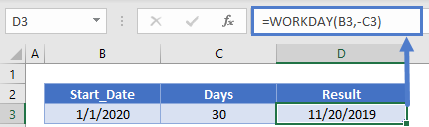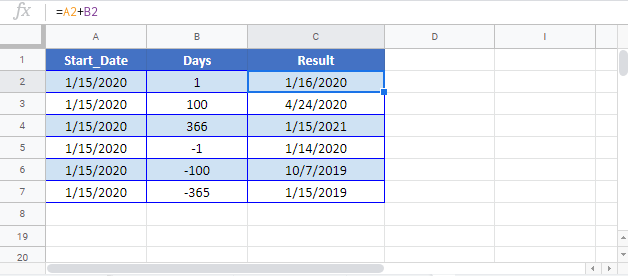# Add or Subtract Days to a Date in Excel & Google Sheets

This tutorial will demonstrate how to add (or subtract) days to a date in Excel and Google Sheets.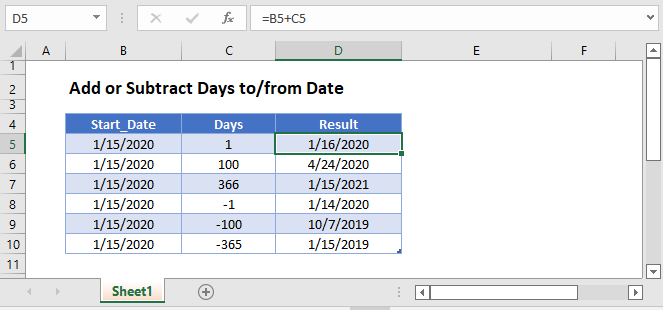## Add Days to a Date

Dates in Excel are stored as serial numbers where each whole number represents a unique day: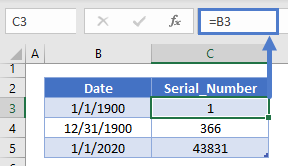This makes adding or subtracting dates extremely easy. Simply add a number of days directly to the date:

``=B3+C3``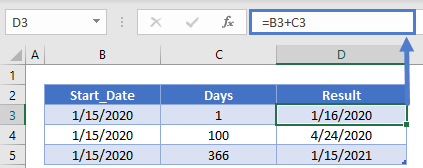## Subtract Days From a Date

Of course, you can subtract days in the same way:

``=B3-C3``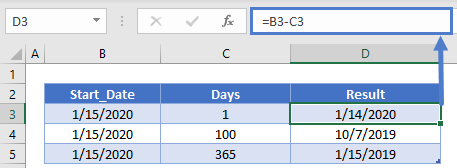Excel also provides you with functions to work with Business Days. Specifically the WORKDAY Function allows you to add business days to a date:

``=WORKDAY(B3,C3)``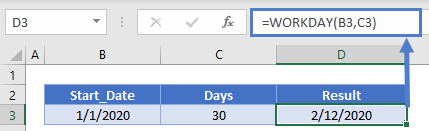### Subtract Working Days

Or you can subtract working days the same way:

``=WORKDAY(B3,-C3)``